### Keyword IRC

This keyword activates the intrinsic reaction coordinate (IRC) calculation. The keyword HESSIAN (see 4.6.5) is mandatory for this keyword.
Options:
FORWARD / REVERSE
 FORWARD The IRC is calculated in the forward direction of the transition state eigenvector of the Hessian. REVERSE The IRC is calculated in the reverse direction of the transition state eigenvector of the Hessian.
MASS / NOMASS
 MASS The IRC is calculated in mass-weighted coordinates. NOMASS The IRC is calculated without mass-weighting coordinates. RESTART The IRC calculation is restarted. Previous steps are read from the file deMon.trj. EXTEND An already-calculated IRC branch is extended. MAX=Integer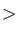Maximum number of IRC steps. Default is 50. STEP=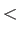RealMaximum radius size for IRC optimization. Default is 0.1 (amu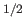)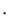Å. TOL=RealConvergence criterion for IRC end-point gradients. Default is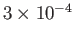atomic units. EVIB=Real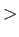Energy threshold for transition state kick-off. Default is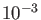Hartree. READ=IntegerNumber of IRC pivot points for which coordinates are read from the file deMon.trj.
Description:
Calculation of the intrinsic reaction coordinate (IRC) yields the connectivity among reactant, transition state, and product on the potential energy surface . Only with this connectivity may a reaction be broken down into its elementary steps. IRC calculations are also useful to discover unexpected intermediates that are often overlooked in the transition state search. In deMon2k, IRC calculations are performed with the Gonzalez-Schlegel algorithm [202,203]. Each IRC step possesses two stages. First is the generation of a pivot point. Second is constrained optimization on a hypersphere around this point. With the option STEP, the (maximum) radius of this hypersphere is defined. The IRC path is divided into two branches, one for the forward direction of the transition state eigenvector (which is the eigenvector that corresponds to the imaginary frequency of the transition state), and the other for the reverse direction of this eigenvector . Thus, the IRC calculation always starts at the transition state structure and needs the Hessian matrix for this structure. As a result the keyword HESSIAN is mandatory for an IRC input. It is convenient to use the restart Hessian from the frequency analysis of the optimized transition state structure. To do so the deMon.mem file from the transition state frequency analysis must be copied to the deMon.rst file for the IRC calculation and HESSIAN RESTART must be specified in the IRC input file. Note that the structure definition for such an IRC calculation must coincide with the one in the deMon.new file from the transition state frequency analysis. The IRC keyword options FORWARD and REVERSE are used to specify IRC calculations along the forward and reverse direction of the Hessian eigenvector that corresponds to the imaginary frequency of the transition state (please note that eigenvector directions may change on account of variations in diagonalization routines and computer architectures). The two branches can be merged with the option EXTEND. Assuming that an IRC FORWARD calculation was already performed (see exampleon pageof the tutorial), the following input extends the IRC path with the REVERSE branch:

 IRC REVERSE EXTEND


As a result, the trajectory and molden files deMon.trj and deMon.mol contain both branch-
es and, thus, the full reaction coordinate. Coordinates of a specified pivot point along the IRC path can be loaded with the READ option of the IRC keyword. In this way, properties or molecular fields can be calculated or plotted along the IRC path.

By default, the IRC path is calculated in mass-weighted coordinates. The mass-weighting is disabled with the option NOMASS. With the MAX option of the IRC keyword, the maximum number of IRC steps can be specified. The RESTART option permits the restart of an IRC calculation, e.g. if not enough IRC steps were requested in a previous run. The IRC calculation restarts with the last successfully calculated pivot point. As always, the restart input can be found in the deMon.new file. For the IRC restart, the file deMon.mem, must be renamed to deMon.rst, and the file deMon.trj must also be available. The TOL option specifies the convergence criterion for the RMS gradient at the IRC path end points. The convergence of the constrained optimizations in each IRC step can be modified with the OPTIMIZATION keyword. Cartesian constants can be used in an IRC calculation. With the EVIB option, the energy decrease for the transition state kick-off (that is, the energy decrement to initiate the search) along the transition state eigenvector is specified. This energy value is given in Hartree.